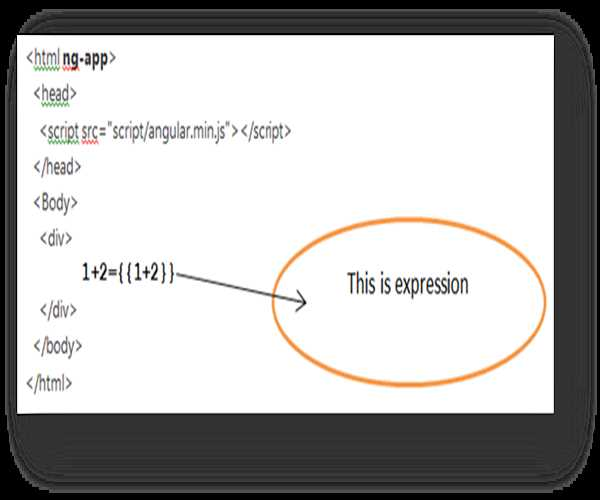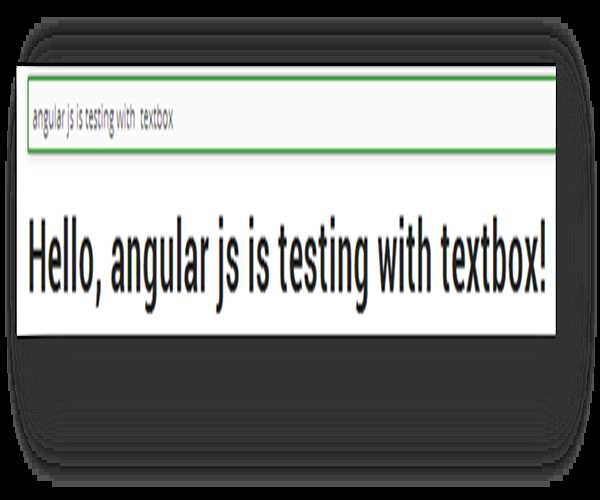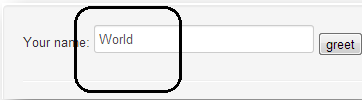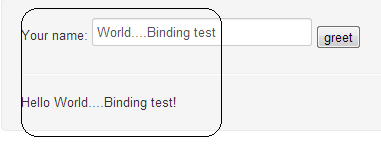I would like to introduce basics of Angularjs, I collected following information from various web resources and tried to explain in easy way.

•   Angularjs is a Javascript MVC framework created by Google to build properly architecture and maintainable web applications
•   It decreases emphasis on directly handling DOM manipulation from the application logic
•   It employs efficient two-way data binding and sensible MVC implementation.
•   We can create our own HTML custom tags and attributes
•   Angularjs revolves around Model, view and Controller
•   Using Angularjs we can get rid of from document.getelemetbyid(‘..’) etc.

Before moving ahead I am assuming we are aware about MVC framework.

##### We will learn following things in AngularJs

a)      Directives

b)      Expressions

c)       \$scope object

d)      Controllers

e)      Model

f)       Module

g)      Filters

h)      Data binding

Following is the Explanation of above listed things.

1)      Directives:

Directives can be used to create custom HTML tags that serve as new, custom widgets. They can also be used to “decorate” elements with behavior and manipulate DOM attributes in interesting ways. The HTML design static pages now we use extended HTML which provides dynamic feature by adding attribute, element or comments.

We generally have directive starting with ng-.

We need to add directive to existing HTML form “np-app”

<html ng-app>

We have following directives which are below

a)      ng-app  :

This will activate the AngularJS to the entire document

We need to add in HTML form like this : <html  ng-app>

b)      ng-model:

This links the model and the form. This mean that any changes to the

control update the data in our model and when we update the      model it updates the control. We can define model by this way:

<input ng-model="modeltest">

c)       ng-controllers :

In Angular, a Controller is a JavaScript constructor function that is used to augment the Angular Scope.

<div ng-controller="modeltest">

Here we have to define controller having name “modeltest” in separate javascript file.

Other directives are below:

d)      ng-click

e)      ng-repeat

f)       ng-view

g)      ng-bind

We have different ways to define directive and all of below have same meaning

<input ng-model="modeltest">

<input data-ng:model=" modeltest ">

<input ng:model=" modeltest ">

<input ng_model=" modeltest ">

2)      Expressions :

a)      The {{   }} are a declarative way of specifying data binding location in the HTML. AngularJS will automatically update this text.

b)      Using expression we can do data binding, as in {{ }}

 Expression example##### Output of above HTML

Following Output will be will be render on DOM

1+2=3

##### Html file
```<html ng-app>

<script src="script/angular.min.js"></script>

<body>

<div>

<input type="text" ng-model="uservalue" placeholder="enter text here">

<h1>Hello, {{ uservalue }}</h1>

</div>

</body>

</html>```

From above code will try to understand following terms

a)      ng-model: it is model directive here which can be bi-directional. Here model name is uservalue

b)      {{ uservalue }} : {{ }}  it is expressions in Angularjs and uservalue inside {{ }} is to  be display on HTML form.

c)       Following is output of above html code.

 HTML output4)      Angular Module

An Angular module is simply a collection of functions defined in javascript file.

Steps to define module in angularjs

a) First need to create sample.js

b) Inside sample.js file we need to add following line

var app = angular.module('myApp', []);

This line creates the Angular module named “myApp”.

c) We need to define this module in our DOM, module name is “myApp”

d) <html ng-app="myApp">

5)      Controllers

In Angular, a Controller is a JavaScript constructor function that is used to augment the Angular Scope.

We have a code below in table, left column contains html code and right column contains Sample.js. Here we are defining MyUserController in Sample.js. We can define controller in HTML form using “ng-controller” directive.

##### HTML file
```<!doctype html>

<html ng-app>

<script src="angular.min.js"></script>

<script src="sample.js"></script>

<body>

<div ng-controller="MyUserController">

<input type="text" ng-model="name">

<button ng-click='sayHello()'>greet</button>

<hr>

{{greeting}}

</div>

</body>

</html>```

Sample.js

```function MyUserController (\$scope) {

\$scope.name = 'World';

\$scope.sayHello = function() {

\$scope.greeting = 'Hello ' + \$scope.name + '!';

};

}```

Here in above example, MyUserController is a function defined in sample.js and this function has an input parameters \$scope. First we will learn about \$scope and then try to understand above example.

6)     \$scope object

•  Scope is the glue between application controller and the view.
•  A \$scope is an object that ties a view (a DOM element) to the controller.
•  In the Model-View-Controller structure, this \$scope object becomes the model.
•  The \$scope is just a JavaScript object. Both the controller and the view have access to the \$scope so it can be used for communication between the two.

 Relationship of \$scope with View and controller7)      Going back to point 5.  Following is the screen will be rendered on DOM.

 Before clicking greet button After clicking greet buttonFollowing is the description of above example

a)      From left column  we are getting “World” in text box is only due to \$scope binding done at controller

\$scope.name = ‘World’ at controller and ng-model="name" at DOM

(This is what we can say one way binding)

b)       From right column, here I typed a text and then clicked on greet button and I get “Hello World....Binding test!” on DOM. It is due to declaring {{ greeting }} at DOM (it is an expression) and\$scope.greeting = 'Hello ' + \$scope.name + '!' at MyUserController function.

c)       Here we have two way binding using\$scope.name and ng-model="name” which is defined at DOM and Controller as well. We got default value at text box when DOM loaded and are getting updated value of username when we clicked on button.

8)      Filters

Filters allow formatting the data, which to be display on DOM.

Filters are invoked in the HTML with the | (pipe) character inside expressions {{ }}.

Few examples

 Filter Filter with Expression Output at DOM Currency {{ 123 | currency }} \$123.00 Currency {{ 456 | currency:'USD \$' }} USD \$456.00 Date {{ today | date:'MMM d, y' }} Nov 20, 2013 Number {{ 1234567890 | number }} 1,234,567,890 Uppercase {{ "csharp" | uppercase }} CSHARP String {{ ['Ari', 'Likes', 'To']  | filter:'e' }} Likes

Modified On Sep-07-2019 12:14:40 AM
1.nice article